# Hann-Poisson window

The Hann-Poisson window coefficients are given by the following formula

$$a(k)=0.5 * (1-\cos(\frac{\pi\,k}{M}))\,e^{-\alpha\frac{|k-M|}{M}}$$

where N is the length of the filter, M = (N – 1) / 2, k = 0, 1, …, N – 1, and usually 0 ≤ α < 1.

The Hann-Poisson window is the product of the Hann window and the Poisson window.

## An example Hann-Poisson window

Consider a finite impulse response (FIR) low pass filter of length N = 201. The following is the Hann-Poisson window with α = 0.3.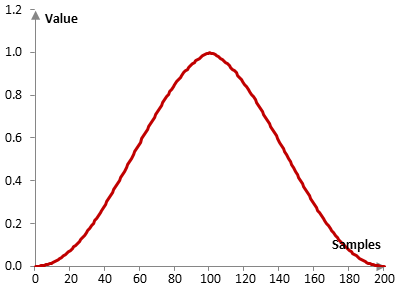Given a sampling frequency of 2000 Hz and a filter cutoff frequency of 40 Hz, the impulse response of the filter with a rectangular window (with no window) and with the Hann-Poisson window is as follows.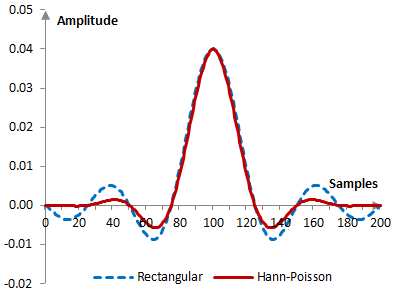The magnitude response of the same filter is shown on the graph below.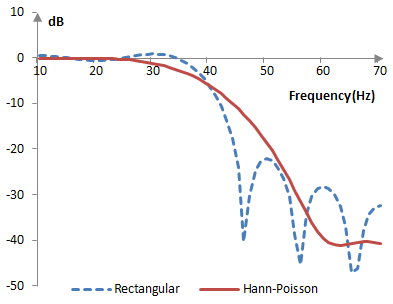As the parameter α becomes larger, the transition band of the Hann-Poisson window increases and its Gibbs phenomenon ripples become smaller.

The following is the Hann-Poisson window with N = 201 and at three different values of α (0.3, 0.5, and 0.7).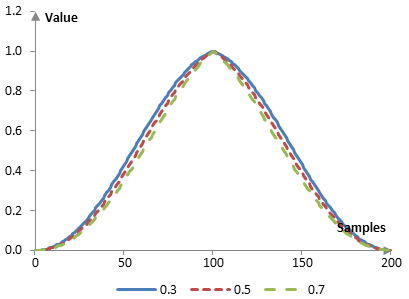The magnitude response of a filter at these values of α, given a sampling frequency of 2000 Hz and a cutoff frequency of 40 Hz, is shown below.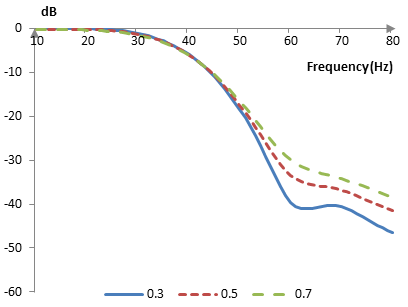## Measures for the Hann-Poisson window

The following is a comparison of the discrete Fourier transform of the Hann-Poisson window and the rectangular window.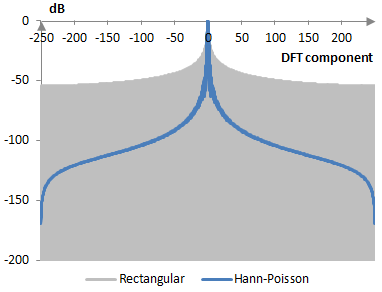The Hann-Poisson window measures are as follows.

 α 0.3 0.5 0.7 Coherent gain 0.46 0.43 0.41 Equivalent noise bandwidth 1.57 1.61 1.66 Processing gain -1.95 dB -2.07 dB -2.20 dB Scalloping loss -1.32 dB -1.25 dB -1.19 dB Worst case processing loss -3.27 dB -3.33 dB -3.40 dB Highest sidelobe level -37.6 dB -35.2 dB -33.0 dB Sidelobe falloff -17.4 dB / octave, -57.7 dB / decade -16.8 dB / octave, -55.9 dB / decade -16.4 dB / octave, -54.6 dB / decade Main lobe is -3 dB 1.50 bins 1.54 bins 1.58 bins Main lobe is -6 dB 2.08 bins 2.14 bins 2.20 bins Overlap correlation at 50% overlap 0.140 0.124 0.110 Amplitude flatness at 50% overlap 0.861 0.779 0.705 Overlap correlation at 75% overlap 0.631 0.612 0.591 Amplitude flatness at 75% overlap 0.977 0.960 0.942

Window

admin: First posted on 2014 04 20

mic, 2014 04 20: I've just fixed this topic. The previous version of the topic was wrong - it was missing a minus before the alpha parameter. the window still worked, but it wasn't the Hann-Poisson window.

### Filtered HTML

• Freelinking helps you easily create HTML links. Links take the form of [[indicator:target|Title]]. By default (no indicator): Click to view a local node.